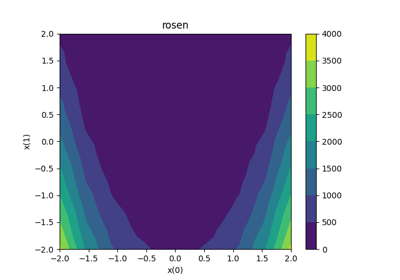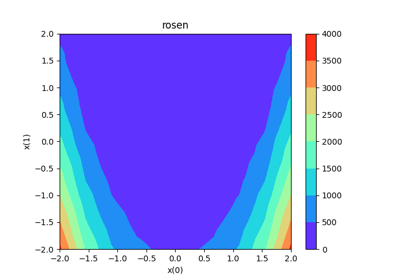gemseo / post / dataset

# zvsxy module¶

Draw a variable versus two others from a Dataset.

A ZvsXY plot represents the variable $$z$$ with respect to $$x$$ and $$y$$ as a surface plot, based on a set of points :points $$\{x_i,y_i,z_i\}_{1\leq i \leq n}$$. This interpolation is relies on the Delaunay triangulation of $$\{x_i,y_i\}_{1\leq i \leq n}$$

class gemseo.post.dataset.zvsxy.ZvsXY(dataset, x, y, z, x_comp=0, y_comp=0, z_comp=0, add_points=False, fill=True, levels=None, other_datasets=None)[source]

Bases: DatasetPlot

Plot surface z versus x,y.

Parameters:
• dataset (Dataset) – The dataset containing the data to plot.

• x (str) – The name of the variable on the x-axis.

• y (str) – The name of the variable on the y-axis.

• z (str) – The name of the variable on the z-axis.

• x_comp (int) –

The component of x.

By default it is set to 0.

• y_comp (int) –

The component of y.

By default it is set to 0.

• z_comp (int) –

The component of z.

By default it is set to 0.

Whether to display the entries of the dataset as points above the surface.

By default it is set to False.

• fill (bool) –

Whether to generate a filled contour plot.

By default it is set to True.

• levels (int | Sequence[int]) – Either the number of contour lines or the values of the contour lines in increasing order. If None, select them automatically.

• other_datasets (Iterable[Dataset]) – Additional datasets to be plotted as points above the surface.

Raises:

ValueError – If the dataset is empty.

execute(save=True, show=False, file_path=None, directory_path=None, file_name=None, file_format=None, properties=None, fig=None, axes=None, **plot_options)

Execute the post processing.

Parameters:
• save (bool) –

If True, save the plot.

By default it is set to True.

• show (bool) –

If True, display the plot.

By default it is set to False.

• file_path (str | Path | None) – The path of the file to save the figures. If None, create a file path from directory_path, file_name and file_format.

• directory_path (str | Path | None) – The path of the directory to save the figures. If None, use the current working directory.

• file_name (str | None) – The name of the file to save the figures. If None, use a default one generated by the post-processing.

• file_format (str | None) – A file format, e.g. ‘png’, ‘pdf’, ‘svg’, … If None, use a default file extension.

• properties (Mapping[str, DatasetPlotPropertyType] | None) – The general properties of a DatasetPlot.

• fig (None | Figure) – The figure to plot the data. If None, create a new one.

• axes (None | Axes) – The axes to plot the data. If None, create new ones.

• **plot_options – The options of the current class inheriting from DatasetPlot.

Returns:

The figures.

Raises:

AttributeError – When the name of a property is not the name of an attribute.

Return type:

list[Figure]

color: str | list[str]

The color(s) for the series.

If empty, use a default one.

colormap: str

The color map.

dataset: Dataset

The dataset to be plotted.

fig_size: tuple[float, float]

The figure size.

property fig_size_x: float

The x-component of figure size.

property fig_size_y: float

The y-component of figure size.

font_size: int

The font size.

property labels: Mapping[str, str]

The labels of the variables.

legend_location: str

The location of the legend.

linestyle: str | list[str]

The line style(s) for the series.

If empty, use a default one.

marker: str | list[str]

The marker(s) for the series.

If empty, use a default one.

property output_files: list[str]

The paths to the output files.

title: str

The title of the plot.

xlabel: str

The label for the x-axis.

xmax: float | None

The maximum value on the x-axis.”

If None, compute it from data.

xmin: float | None

The minimum value on the x-axis.

If None, compute it from data.

ylabel: str

The label for the y-axis.

ymax: float | None

The maximum value on the y-axis.

If None, compute it from data.

ymin: float | None

The minimum value on the y-axis.

If None, compute it from data.

zlabel: str

The label for the z-axis.

zmax: float | None

The maximum value on the z-axis.

If None, compute it from data.

zmin: float | None

The minimum value on the z-axis.

If None, compute it from data.

## Examples using ZvsXY¶Plot - ZvsXY

Plot - ZvsXYRosenbrock dataset

Rosenbrock dataset